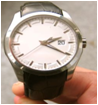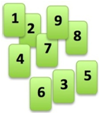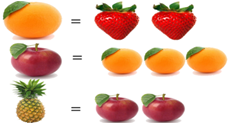# SOF - IMO PDF Sample Papers for Class 3

Class 3 sample paper & practice questions for International Mathematics Olympiad (IMO) level 1 are given below. Syllabus for level 1 is also mentioned for these exams. You can refer these sample paper & quiz for preparing for the exam.#### Resources:

##### Sample Questions from Olympiad Success:
 Q.1 Q.2 Q.3 Q.4 Q.5 Q.6 Q.7 Q.8 Q.9 Q.10
 Q.1 What time will the analog wristwatch show in 45 minutes?a) 15:45 b) 2:65 c) 3:05 d) 2:45
 Q.2 83,040 toys sold during 12 days of fair. If the equal number of toys were sold every day, then find the number of toys sold in one day: a) 3040 b) 5304 c) 6920 d) 5920
 Q.3 A number has 7 at the tens place. There is a zero in the thousands place. The number 5 is at the hundreds place. The number 5 is at the ones place. There is a number 1 at the ten thousand places. What is the number?a) 10,557 b) 10,575 c) 15,075 d) 51,075
 Q.4 Which among the following is less and by how much? (75.16 + 12.2) or (500.12 - 412.78) a) (500.12 - 412.78) is greater and the difference is Rs. 0.2 b) (500.12 - 412.78) is greater and the difference is 2 paise c) (75.16 + 12.2) is greater and the difference is Rs. 0.2 d) (75.16 + 12.2) is greater and the difference is 2 paise
 Q.5 Find the difference between the largest possible even number and smallest possible odd number from given digits:6, 5, 8, 9, 3.   (IMO L2 2014) a) 62,847 b) 62,964 c) 62,874 d) 62,946
 Q.6 Rahul has five notes of rupees twenty, six notes of rupees ten, three notes of rupees five and two notes of rupees fifty. If he bought a toy of rupees two hundred and eighteen, then how much money does he have now? a) Rs. 57 b) Rs. 55 c) Rs. 59 d) Rs. 47
 Q.7 If each strawberry weighs 37 g, then find the weight of pineapple according to the relation given in the image:a) 404 g b) 454 g c) 424 g d) 444 g
 Q.8 A number is less than 50 and a common multiple of  6 and 8. The sum of its digits is 6. What is the number? a) 15 b) 42 c) 24 d) 48
 Q.9 Arrange these numbers from the greatest to the smallest:56, 3 × 8, 29, 2 × 7, 2, 19.   (IMO L2 2012) a) 56, 2 × 7, 3 × 8, 29, 2, 19. b) 2, 2 × 7, 19, 3 × 8, 29, 56. c) 56, 29, 3 × 8, 19, 2 × 7, 2. d) 2 × 7, 3 × 8, 29, 2, 19, 56.
 Q.10 A sugarcane field requires 15 L of water per day and banana field requires 45 L of water per day for irrigation. What is the total quantity of water required for irrigation in a month? a) 1350 L b) 1380 L c) 1800 L d) 450 LSample PDF of SOF - International Mathematics Olympiad (IMO) PDF Sample Papers for Class 3:

 Q.1 )c Q.2 )c Q.3 )b Q.4 )d Q.5 )a Q.6 )a Q.7 )d Q.8 )c Q.9 )c Q.10 )c

Q.1 : c | Q.2 : c | Q.3 : b | Q.4 : d | Q.5 : a | Q.6 : a | Q.7 : d | Q.8 : c | Q.9 : c | Q.10 : c